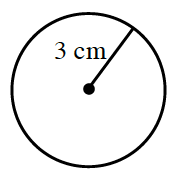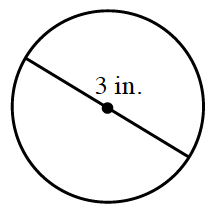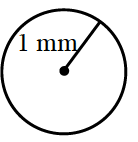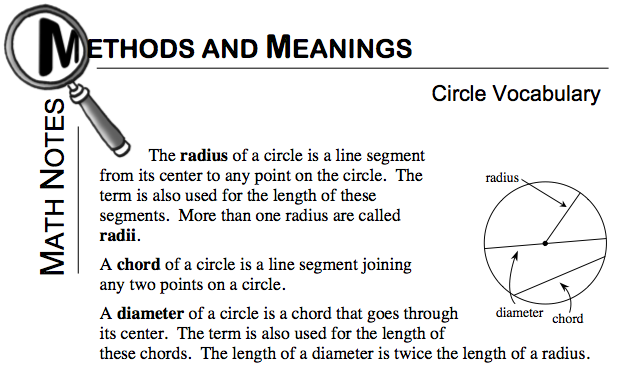### Home > CCAA > Chapter 8 Unit 7 > Lesson CC2: 8.3.3 > Problem8-87

8-87.

Read the Math Notes box for this lesson and then find the length of the radius and diameter of each of the following circles.  Homework Help ✎

Refer to the Math Notes box below in order to answer this question.

1.Radius: $3$ cm
Diameter: $6$ cm

1.How is the information for this circle different than part (a)?

Radius: $1.5$ in
Diameter: $3$ in

1.Use the same method as part (a).